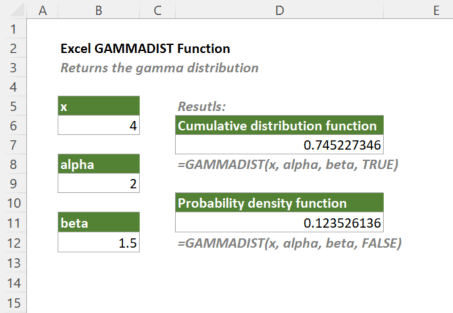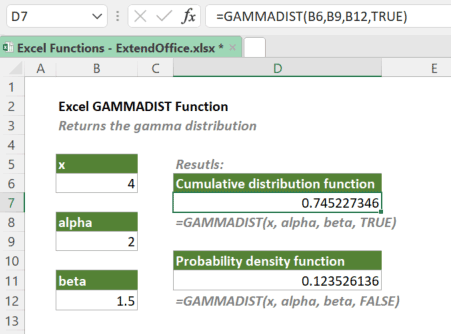The GAMMADIST function returns the gamma distribution. The function is frequently used to provide probabilities for values that may have a skewed distribution, such as queuing analysis.

Note: The GAMMADIST function has been replaced with GAMMA.DIST. Although GAMMADIST is still available in current versions of Excel, you should consider using the new function from now on, because GAMMADIST may not be available in Excel’s future versions.#### Arguments

• x (required): The value at which you want to calculate the distribution. Note: x must be greater than or equal to 0.
• alpha (required): A parameter of the distribution. Note: alpha must be positive.
• beta (required): A parameter of the distribution. If beta = 1, then the standard gamma distribution is calculated. Note: beta must be positive.
• cumulative (required): A logical argument that specifies the type of gamma distribution to be calculated:
• TRUE: Calculate the cumulative distribution function;
• FALSE: Calculate the probability density function.

#### Return Value

The GAMMADIST function returns a numeric value.

#### Function Notes

• GAMMADIST returns the #NUM! error if any of the below conditions is met:
• x < 0;
• alpha ≤ 0;
• beta ≤ 0.
• GAMMADIST returns the #VALUE! error if:
• x, alpha, or beta is not a numeric value;
• cumulative is not recognized as a logical value.

#### Example

To calculate both the cumulative distribution function and the probability density function for given x, alpha and beta values, you should set the cumulative argument as TRUE and FALSE respectively. Please copy or enter the formulas below in the result cells and then press Enter to get the results.Also, you can type the actual x, alpha and beta values in the formulas as shown below.

The Excel GAMMAINV function is the inverse of the GAMMADIST function (when used to calculate the cumulative distribution function, i.e., the cumulative argument is set to TURE). For a supplied value for x, GAMMADIST(x, alpha, beta, TRUE) returns the value of probability such that x = GAMMAINV(probability, alpha, beta).

#### Related functions

Excel GAMMA.DIST Function

The GAMMA.DIST function returns the gamma distribution. The function is frequently used to provide probabilities for values that may have a skewed distribution, such as queuing analysis.

Excel GAMMA Function

The GAMMA function returns the value of the gamma function for a specified number.

Excel GAMMAINV Function

The GAMMAINV function returns the inverse of the gamma cumulative distribution. The function is frequently used to provide probabilities for values that may have a skewed distribution, such as queuing analysis.

### The Best Office Productivity Tools

#### Kutools for Excel - Helps You To Stand Out From Crowd

 Popular Features: Find, Highlight or Identify Duplicates  |  Delete Blank Rows  |  Combine Columns or Cells without Losing Data  |  Round without Formula ... Super VLookup: Multiple Criteria  |  Multiple Value  |  Across Multi-Sheets  |  Fuzzy Lookup... Adv. Drop-down List: Easy Drop Down List  |  Dependent Drop Down List  |  Multi-select Drop Down List... Column Manager: Add a Specific Number of Columns  |  Move Columns  |  Toggle Visibility Status of Hidden Columns  |  Compare Columns to Select Same & Different Cells ... Featured Features: Grid Focus  |  Design View  |  Big Formula Bar  |  Workbook & Sheet Manager | Resource Library (Auto Text)  |  Date Picker  |  Combine Worksheets  |  Encrypt/Decrypt Cells  |  Send Emails by List  |  Super Filter  |  Special Filter (filter bold/italic/strikethrough...) ... Top 15 Toolsets:  12 Text Tools (Add Text, Remove Characters ...)  |  50+ Chart Types (Gantt Chart ...)  |  40+ Practical Formulas (Calculate age based on birthday ...)  |  19 Insertion Tools (Insert QR Code, Insert Picture from Path ...)  |  12 Conversion Tools (Numbers to Words, Currency Conversion ...)  |  7 Merge & Split Tools (Advanced Combine Rows, Split Excel Cells ...)  |  ... and more

Kutools for Excel Boasts Over 300 Features, Ensuring That What You Need is Just A Click Away...#### Office Tab - Enable Tabbed Reading and Editing in Microsoft Office (include Excel)

• One second to switch between dozens of open documents!
• Reduce hundreds of mouse clicks for you every day, say goodbye to mouse hand.
• Increases your productivity by 50% when viewing and editing multiple documents.
• Brings Efficient Tabs to Office (include Excel), Just Like Chrome, Edge and Firefox.